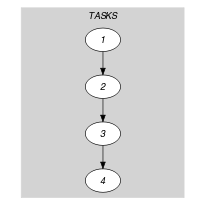## 5.326. precedence

Origin

Scheduling

Constraint

$\mathrm{𝚙𝚛𝚎𝚌𝚎𝚍𝚎𝚗𝚌𝚎}\left(\mathrm{𝚃𝙰𝚂𝙺𝚂}\right)$

Argument
 $\mathrm{𝚃𝙰𝚂𝙺𝚂}$ $\mathrm{𝚌𝚘𝚕𝚕𝚎𝚌𝚝𝚒𝚘𝚗}\left(\mathrm{𝚘𝚛𝚒𝚐𝚒𝚗}-\mathrm{𝚍𝚟𝚊𝚛},\mathrm{𝚍𝚞𝚛𝚊𝚝𝚒𝚘𝚗}-\mathrm{𝚍𝚟𝚊𝚛}\right)$
Restrictions
 $\mathrm{𝚛𝚎𝚚𝚞𝚒𝚛𝚎𝚍}$$\left(\mathrm{𝚃𝙰𝚂𝙺𝚂},\left[\mathrm{𝚘𝚛𝚒𝚐𝚒𝚗},\mathrm{𝚍𝚞𝚛𝚊𝚝𝚒𝚘𝚗}\right]\right)$ $\mathrm{𝚃𝙰𝚂𝙺𝚂}.\mathrm{𝚍𝚞𝚛𝚊𝚝𝚒𝚘𝚗}\ge 0$
Purpose

All consecutive pairs of tasks of the collection $\mathrm{𝚃𝙰𝚂𝙺𝚂}$ should be ordered (i.e., the end of the first task of a pair should be less than or equal to the start of the second task of the same pair).

Example
$\left(\begin{array}{c}〈\begin{array}{cc}\mathrm{𝚘𝚛𝚒𝚐𝚒𝚗}-1\hfill & \mathrm{𝚍𝚞𝚛𝚊𝚝𝚒𝚘𝚗}-3,\hfill \\ \mathrm{𝚘𝚛𝚒𝚐𝚒𝚗}-4\hfill & \mathrm{𝚍𝚞𝚛𝚊𝚝𝚒𝚘𝚗}-0,\hfill \\ \mathrm{𝚘𝚛𝚒𝚐𝚒𝚗}-5\hfill & \mathrm{𝚍𝚞𝚛𝚊𝚝𝚒𝚘𝚗}-2,\hfill \\ \mathrm{𝚘𝚛𝚒𝚐𝚒𝚗}-8\hfill & \mathrm{𝚍𝚞𝚛𝚊𝚝𝚒𝚘𝚗}-1\hfill \end{array}〉\hfill \end{array}\right)$

Since the tasks are ordered (i.e., $1+3\le 4$, $4+0\le 5$, $5+2\le 8$) the $\mathrm{𝚙𝚛𝚎𝚌𝚎𝚍𝚎𝚗𝚌𝚎}$ constraint holds.

Typical
 $|\mathrm{𝚃𝙰𝚂𝙺𝚂}|>2$ $\mathrm{𝚃𝙰𝚂𝙺𝚂}.\mathrm{𝚍𝚞𝚛𝚊𝚝𝚒𝚘𝚗}\ge 1$
Symmetries
• $\mathrm{𝚃𝙰𝚂𝙺𝚂}.\mathrm{𝚍𝚞𝚛𝚊𝚝𝚒𝚘𝚗}$ can be decreased to any value $\ge 0$.

• One and the same constant can be added to the $\mathrm{𝚘𝚛𝚒𝚐𝚒𝚗}$ attribute of all items of $\mathrm{𝚃𝙰𝚂𝙺𝚂}$.

Arg. properties

Contractible wrt. $\mathrm{𝚃𝙰𝚂𝙺𝚂}$.

Keywords
Arc input(s)

$\mathrm{𝚃𝙰𝚂𝙺𝚂}$

Arc generator
$\mathrm{𝑃𝐴𝑇𝐻}$$↦\mathrm{𝚌𝚘𝚕𝚕𝚎𝚌𝚝𝚒𝚘𝚗}\left(\mathrm{𝚝𝚊𝚜𝚔𝚜}\mathtt{1},\mathrm{𝚝𝚊𝚜𝚔𝚜}\mathtt{2}\right)$

Arc arity
Arc constraint(s)
$\mathrm{𝚝𝚊𝚜𝚔𝚜}\mathtt{1}.\mathrm{𝚘𝚛𝚒𝚐𝚒𝚗}+\mathrm{𝚝𝚊𝚜𝚔𝚜}\mathtt{1}.\mathrm{𝚍𝚞𝚛𝚊𝚝𝚒𝚘𝚗}\le \mathrm{𝚝𝚊𝚜𝚔𝚜}\mathtt{2}.\mathrm{𝚘𝚛𝚒𝚐𝚒𝚗}$
Graph property(ies)
$\mathrm{𝐍𝐀𝐑𝐂}$$=|\mathrm{𝚃𝙰𝚂𝙺𝚂}|-1$

Graph model

Since we are only interested by the constraints linking two consecutive items of the collection $\mathrm{𝚃𝙰𝚂𝙺𝚂}$ we use $\mathrm{𝑃𝐴𝑇𝐻}$ to generate the arcs of the initial graph.

Parts (A) and (B) of Figure 5.326.1 respectively show the initial and final graph of the first example of the Example slot. Since we use the $\mathrm{𝐍𝐀𝐑𝐂}$ graph property, the arcs of the final graph are stressed in bold.

##### Figure 5.326.1. Initial and final graph of the $\mathrm{𝚙𝚛𝚎𝚌𝚎𝚍𝚎𝚗𝚌𝚎}$ constraint(a) (b)